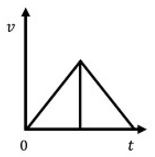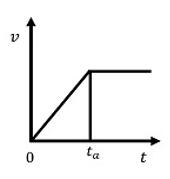These calculators are used to calculate linear motion. If you need to calculate limited angle rotary motion, please use the rotary motion calculators.
Unit Converter
Conversion Type Units Units

## Newton's Second law:

$$F = m \times a$$

Force = Mass x Acceleration

To size a stage properly the force must be known. If force is not known it must be calculated from this equation. The mass is the total mass of the customer payload plus the mass of the moving components of the stage. If the acceleration component is not known it must be calculated. Calculators are provided under the Acceleration tab for estimating the acceleration of a system. Once the force is determined, the duty cycle for all of the specific forces must be determined to calculate the RMS force, which is the average required force. The Force RMS tab provides the tools needed to determine the RMS Force of a motion profile.

Variable Description Units Calculation
m Moving mass
a Acceleration
F Force 0
*accelerations up to 10 g's are possible under closed loop control
*accelerations up to 20 g's are possible under open loop control$$\Large \alpha = \frac{4 \times d }{t^2 \times G}$$
Variable Description Units Calculation
d Distance moved
t Time to complete move (sec)
G Gravitational constant
a Acceleration (g's) 0
*accelerations up to 10 g's are possible under closed loop control
*accelerations up to 20 g's are possible under open loop control$$\Large a = {2 \times d \over t_{a}^2 \times G}$$
Known Variables
Time and Distance are known
Velocity and Distance are known
Time and Velocity are known

Variable Description Units Calculation
d Distance moved
t Time to complete move (sec)
v Velocity
G Gravitational constant
a Acceleration (g's) 0
*accelerations up to 10 g's are possible under closed loop control
*accelerations up to 20 g's are possible under open loop control$$\Large a = {2 \pi^2f^2 D \over G}$$
Known Variables
Frequency and Distance are known
Velocity and Frequency are known
Distance and Velocity are known

Variable Description Units Calculation
d Distance moved
f Frequency (Hz)
v Velocity
G Gravitational constant
a Acceleration (g's) 0
*accelerations up to 10 g's are possible under closed loop control
*accelerations up to 20 g's are possible under open loop control$$\Large F_{rms} = \sqrt{\left ( F_{a} \right )^2 \times t_{a} + \left ( F_{c} \right )^2 \times t_{c} + \left ( F_{d} \right )^2 \times t_{d} \over \left ( t_{on} + t_{off} \right )}$$

Variable Description Units Calculation
Fa Acceleration Force
Fc Constant Velocity Force
Fd Deceleration Force
ta Time to accelerate (sec)
tc Time at constant velocity (sec)
td Time to decelerate (sec)
ton ta + tc + td (sec)
toff Dwell time (sec)
Frms Average required force 0
$$\Large Duty Cycle\left ( \% \right ) ={ t_{on} \over \left ( t_{on} + t_{off}\right )} \times 100$$
Variable Description Calculation
ton Time with power applied (sec)
toff Dwell time (sec)
Duty Cycle (%) Percentage of total time spent active 0
Example
Duty Cycle = 1 sec on, 3 sec off

Duty Cycle = 1/(1+3) = 1/4

Duty Cycle = 25%
Note: Duty Cycle is only for DC motors.
$$\Large F@100\% = Force At DutyCycle \div \sqrt{ 1 \over DutyCycle}$$
Variable Calculation
Duty Cycle %
Force at Duty Cycle
Force at 100 % 0
Example
Force at 10% Duty Cycle = 1 lb X (1/10%)1/2

Force at 10% Duty Cycle = 1 lb X 3.16

Force at 10% Duty Cycle = 3.16 lbs
Note: This calculation is only for DC motors.
Use the following formula for AC motors:
AC Duty Cycle Calculations
Force @ 50% = Force @ 100% * 1.75
Force @ 15% = Force @ 100% * 5
Force @ 3% = Force @ 100% * 8